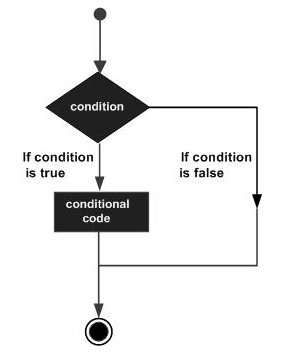# Jython - Decision Control

Decision making structures have one or more conditions to be evaluated or tested by the program, along with a statement or statements that are to be executed, if the condition is determined to be true, and optionally, other statements to be executed, if the condition is determined to be false.

The following illustration shows the general form of a typical decision making structure found in most of the programming languages −Jython does not use curly brackets to indicate blocks of statements to be executed when the condition is true or false (as is the case in Java). Instead, uniform indent (white space from left margin) is used to form block of statements. Such a uniformly indented block makes the conditional code to be executed when a condition given in ‘if’ statement is true.

A similar block may be present after an optional ‘else’ statement. Jython also provides the elif statement using which successive conditions can be tested. Here, the else clause will appear last and will be executed only when all the preceding conditions fail. The general syntax of using if..elif..else is as follows.

```if expression1:
statement(s)
elif expression2:
statement(s)
elif expression3:
statement(s)
else:
statement(s)
```

In the following example, if ..elif ..else construct is used to calculate discount on different values of amount input by user.

```discount = 0
amount = input("enter Amount")
if amount>1000:
discount = amount*0.10
elif amount>500:
discount = amount*0.05
else:
discount = 0
print 'Discount = ',discount
print 'Net amount = ',amount-discount
```

The output of above code will be as shown below.

```enter Amount1500
Discount = 150.0
Net amount = 1350.0
enter Amount600
Discount = 30.0
Net amount = 570.0
enter Amount200
Discount = 0
Net amount = 200
```